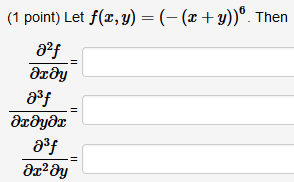# Question Let f(x,y) = (−(x+y))^6. Then: (∂^2f)/(∂x∂y) = (∂^3f)/(∂x∂y∂x) = (∂^3f)/(∂x^2∂y) = (1 point) Let f(x,y) = (-(x+y)). Then 82f 𐐀х у 23f 𐐀хдудх 83f ах2 yLet f(x,y) = (−(x+y))^6. Then:

(∂^2f)/(∂x∂y) =

(∂^3f)/(∂x∂y∂x) =

(∂^3f)/(∂x^2∂y) =Transcribed Image Text: (1 point) Let f(x,y) = (-(x+y)). Then 82f 𐐀х у 23f 𐐀хдудх 83f ах2 y
More
Transcribed Image Text: (1 point) Let f(x,y) = (-(x+y)). Then 82f 𐐀х у 23f 𐐀хдудх 83f ах2 y